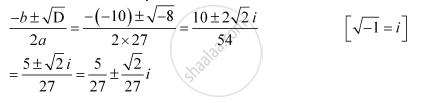CBSE (Arts) Class 11CBSE
Share

# Solve the Quadratic Equation 27x2 – 10x + 1 = 0 - CBSE (Arts) Class 11 - Mathematics

#### Question

Solve the equation 27x2 – 10+ 1 = 0

#### Solution

The given quadratic equation is 27x2 – 10x + 1 = 0

On comparing the given equation with ax2 + bx + c = 0, we obtain

a = 27, b = –10, and c = 1

Therefore, the discriminant of the given equation is

D = b2 – 4ac = (–10)2 – 4 × 27 × 1 = 100 – 108 = –8

Therefore, the required solutions areIs there an error in this question or solution?

#### Video TutorialsVIEW ALL 

Solution Solve the Quadratic Equation 27x2 – 10x + 1 = 0 Concept: Quadratic Equations.
S